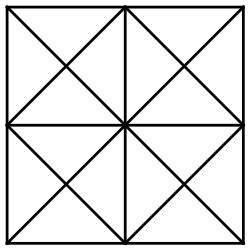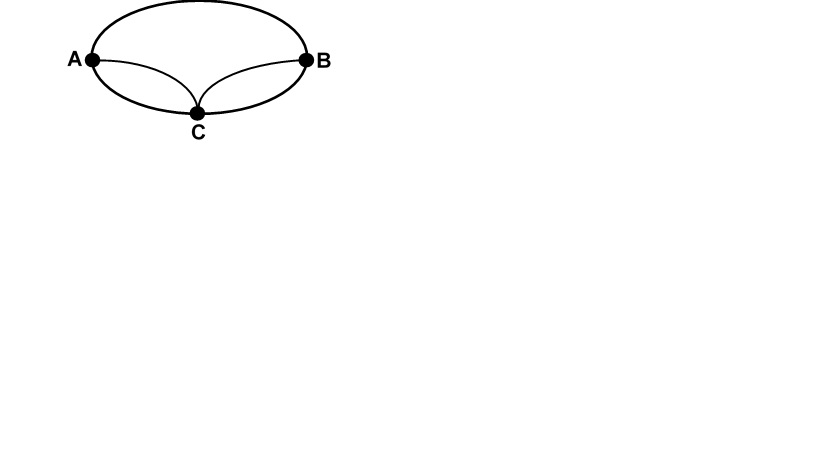# u3a - Maths Challenge - Week 7

Welcome to week 7 of our weekly maths challenge, with problems and puzzles posed by Gordon Burgin, Andrew Holt and the U3A Maths and Stats Subject Adviser - David Martin.

The solutions to week six’s problems are at the bottom of the page. Check back each week for the solutions and let us know how you get on by contacting National Office. New maths puzzles will go up onto the website every Thursday.

### Week Seven

Question 1

I am a two-digit prime number having no divisors other than one and myself. One less than me is a square number while half of one more than me is also a prime number. What am I?

Question 2

Six years ago, Mikayla was 3 times as old as Tatum was. If Mikayla is now 24 years old, how old is Tatum?

Question 3

How many triangles of various sizes are in the figure?Question 4

James has more than 250 toy soldiers. When he tries to put them in rows of 3 there are 2 left over. When he tries to put them in rows of 5 there are 2 left over. When he tries to put them in rows of 7 there are 2 left over. What is the least number of toy soldiers that James may have?

### Week Six Solutions

Question 1

Maura has to visit towns B & C in any order. The roads connecting these towns with her home (A) are shown on the diagram below. How many different routes can she take starting from A and returning to A, going through both B & C (but not more than once through each) and not travelling any road twice on the same trip?Solution
Clockwise: A to B to C (x2) and back to A (x2) = 4 routes,
Counter Clockwise: A to C (x2) to B (x2) and back to A = 4 routes.
Total different routes = 8.

Question 2

I am collecting tokens and already have 12 and collect another 4 each week. When I have 100 I will be able to exchange them for a free meal. When will I be able to do this? A friend has 40 tokens and collects 3 each week. Which of us will be the first to get their free meal?

Solution

After n weeks I will have
4n+12 tokens
For a free meal we require 4n+12 ≥100
Hence n ≥ 22 weeks
After n weeks my friend will have
3n+40 tokens
For a free meal we require 3n+40 ≥ 100
Hence n ≥ 20 weeks
So I will be a couple of weeks behind

Question 3
In the figure below AD = 4, AB = 3, and CD = 9. What is the area of triangle AEC? Note: As a reminder, the area of a triangle is ½ (base x height) and the diagram is not drawn to scale.Solution

If we take AE as the base of triangle AEC, then the height is CD.
The height of triangle AEC is therefore equal to 9 (as given).
To find the base, note that triangles AEB and CDE are similar.
The ratio of AB (3) to CD (9) is 1:3 and is therefore equal to the ratio of AE to ED.
Now dividing AD (4) into this ratio gives us AE = 1 and ED = 3.
The area of triangle AEC would then be = ½ x base (AE) x height (CD) = ½ x 1 x 9 = 4.5.

Question 4
a and b are positive real numbers with a > b which satisfy the equations
ab = 56/(a – b); a2 + b2 = 113/(a – b)
What is a + b?
Solution
(a - b)2 = a2 - 2ab + b2 = (113 – 112)/(a – b) = 1/(a – b)
So (a – b)3 = 1, and hence a = b + 1
Substitute for a in ab = 56/(a – b) to get b(b + 1) = 56/1
Or b2 + b - 56 = 0
(b + 8)(b – 7) = 0
b = 7 (the only valid solution as b is stated to be positive).
Then a = 8, giving a + b = 15.